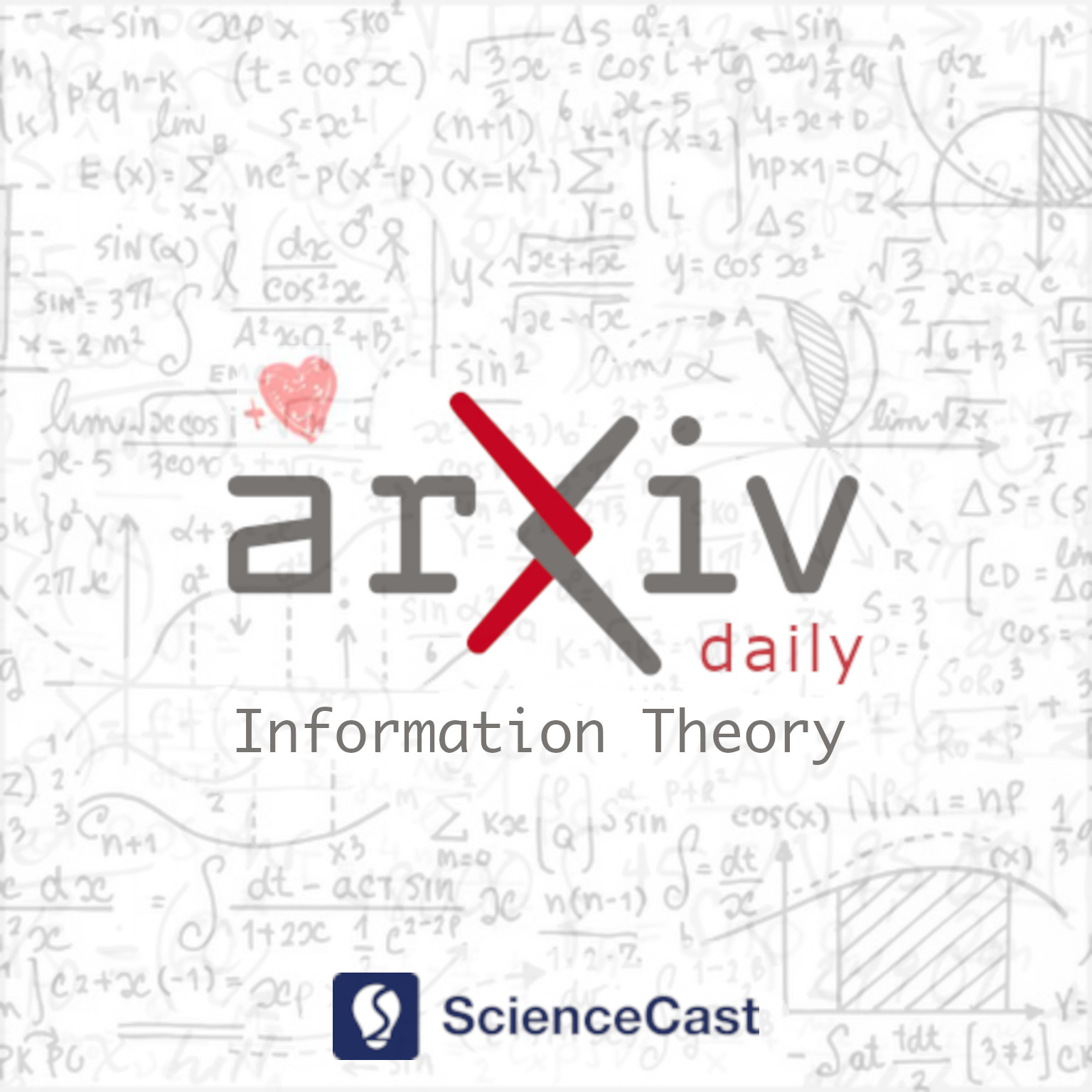##### 1.Sum-rank metric codes

Authors:Elisa Gorla, Umberto Martínez-Peñas, Flavio Salizzoni

Abstract: Sum-rank metric codes are a natural extension of both linear block codes and rank-metric codes. They have several applications in information theory, including multishot network coding and distributed storage systems. The aim of this chapter is to present the mathematical theory of sum-rank metric codes, paying special attention to the $\mathbb{F}_q$-linear case in which different sizes of matrices are allowed. We provide a comprehensive overview of the main results in the area. In particular, we discuss invariants, optimal anticodes, and MSRD codes. In the last section, we concentrate on $\mathbb{F}_{q^m}$-linear codes.

##### 2.Compressed sensing with l0-norm: statistical physics analysis and algorithms for signal recovery

Authors:D. Barbier, C Lucibello, L. Saglietti, F. Krzakala, L. Zdeborova

Abstract: Noiseless compressive sensing is a protocol that enables undersampling and later recovery of a signal without loss of information. This compression is possible because the signal is usually sufficiently sparse in a given basis. Currently, the algorithm offering the best tradeoff between compression rate, robustness, and speed for compressive sensing is the LASSO (l1-norm bias) algorithm. However, many studies have pointed out the possibility that the implementation of lp-norms biases, with p smaller than one, could give better performance while sacrificing convexity. In this work, we focus specifically on the extreme case of the l0-based reconstruction, a task that is complicated by the discontinuity of the loss. In the first part of the paper, we describe via statistical physics methods, and in particular the replica method, how the solutions to this optimization problem are arranged in a clustered structure. We observe two distinct regimes: one at low compression rate where the signal can be recovered exactly, and one at high compression rate where the signal cannot be recovered accurately. In the second part, we present two message-passing algorithms based on our first results for the l0-norm optimization problem. The proposed algorithms are able to recover the signal at compression rates higher than the ones achieved by LASSO while being computationally efficient.

##### 3.How Costly Was That (In)Decision?

Authors:Peng Zou, Ali Maatouk, Jin Zhang, Suresh Subramaniam

Abstract: In this paper, we introduce a new metric, named Penalty upon Decision (PuD), for measuring the impact of communication delays and state changes at the source on a remote decision maker. Specifically, the metric quantifies the performance degradation at the decision maker's side due to delayed, erroneous, and (possibly) missed decisions. We clarify the rationale for the metric and derive closed-form expressions for its average in M/GI/1 and M/GI/1/1 with blocking settings. Numerical results are then presented to support our expressions and to compare the infinite and zero buffer regimes. Interestingly, comparing these two settings sheds light on a buffer length design challenge that is essential to minimize the average PuD.

##### 4.Rectangular Rotational Invariant Estimator for General Additive Noise Matrices

Abstract: Here we consider a problem of multiple measurement vector (MMV) compressed sensing with multiple signal sources. The observation model is motivated by the application of {\em unsourced random access} in wireless cell-free MIMO (multiple-input-multiple-output) networks. We present a novel (and rigorous) high-dimensional analysis of the AMP (approximate message passing) algorithm devised for the model. As the system dimensions in the order, say $\mathcal O(L)$, tend to infinity, we show that the empirical dynamical order parameters -- describing the dynamics of the AMP -- converge to deterministic limits (described by a state-evolution equation) with the convergence rate $\mathcal O(L^{-\frac 1 2})$. Furthermore, we have shown the asymptotic consistency of the AMP analysis with the replica-symmetric calculation of the static problem. In addition, we provide some interesting aspects on the unsourced random access (or initial access) for cell-free systems, which is the application motivating the algorithm.